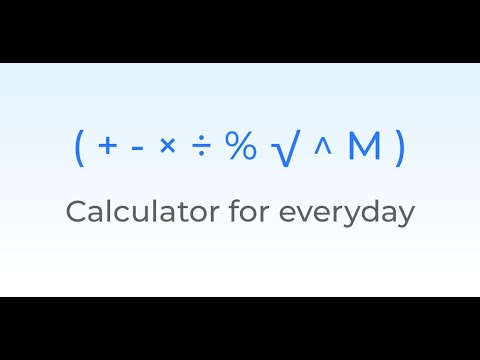Calculator E Plus - Calculator with Memory PlusEveryone
70Calculator E Plus - Everyday Calculator with history save, memory plus function, square root, 00 key and lot more...

A simple, handy and practical calculator with practical functions like a detailed history, power, square root, cube root, memory , etc. useful for everyday calculations and complex expressions.
Get the Digital Calculator new version 2018.

Most suitable for anyone

Calculator FEATURES

1. Simple and easy interface

The Calculator E Plus has interface which is simple to use and powerful as well.
The Calculator Keyboard is specially designed for efficient typing experience.
It has separate keys like ( , ) , power , square root , percentage (%) button and a double-zero ( 00 ) key.

2. Calculator with history. Detailed.

The Calculator E Plus stores detailed history with date and time, so you can search your history to calculate any time. You can edit the calculations in history later or you can copy the calculations to the clipboard.

3. Support for more than 12 digits.

Do limitless calculations with 12 digit calculator. There is no limit on the length of the calculation in this calculator app.
Calculator E Plus supports very big numbers with more than 12 digits.

4. Calculator with Memory Function.

The Calculator has Memory Functions like Memory Plus (M+), Memory Minus (M-), Memory Clear (MC) and Memory Recall (MR). The Calculator E Plus has Memory Keys which allow you to store the results into the Memory variable using M+ , M- , MR , MC keys. The Memory keys work exactly as you want like your standard pro calculator.

5. Supports complex calculations like a pro

Using brackets [ '(' , ')' ] You can calculate any numerical expression of any complexity.
Ex. 100 ÷ ( ( ( 5 + 5 ) + 3 ) + ( 2 + 1 ) x ( 3 x 3 ) ) – 2

6. Calculator with Percentage Button ( % )

You can add or subtract Percentage Value from a number directly using the Percentage ( ' % ' ) key.
% calculator is useful for
- GST Calculator
- VAT Calculator
- Sales tax Calculator
- Margin Calculator
- Tax Calculator
- EMI Calculator
- Interest Calculator
- Solve Mathematics
- Use Memory and Percent for calculations

Example. 100+10%=110

7. Support for variety of operators

The Calculator E Plus supports variety of standard calculator operators.

OPERATIONS you can calculate in Calculator E Plus

Addition ( ' + ' )

Subtraction ( ' - ' )

Multiplication ( ' × ' )

Division ( ' ÷ ' )

Percentage ( ' % ' )

Square Root ( ' √ ' )

Power ( ' ^ ' )

Opening and closing brackets ( ' ( ' ) and ( ' ) ' )

This Calculator app supports all phones powered by Android.
Example,
- Samsung J5 , J6 , J7 , J7 Pro , J7 Prime
- Samsung Galaxy S8 , S8+ , S9 , S9+ , Note8
- Xiomi Mi Note phones
- Xiomi Redmi phones
- Motorola moto e , moto g , moto x , moto z phones
- Google Pixel , Pixel XL , Pixel 2 , Pixel 2 XL
- Huawei phones

Do let us know what do you think about the app. You can review the Calculator E Plus in Google Play or you can directly email us!
Your review will halp us to make the Calculator even better and useful.
Please share the app with your friends!

Thanks!
Collapse

Review Policy
4.5
70 total
5
4
3
2
1

What's New

★ Design Improvements
★ Manually Set Precision
★ Bug Fixes
Collapse

Updated
August 20, 2018
Size
1.1M
Installs
10,000+
Current Version
1.5
Requires Android
4.0 and up
Content Rating
Everyone
Permissions
Offered By